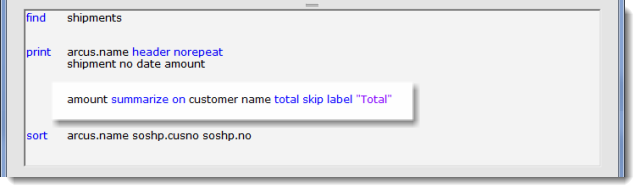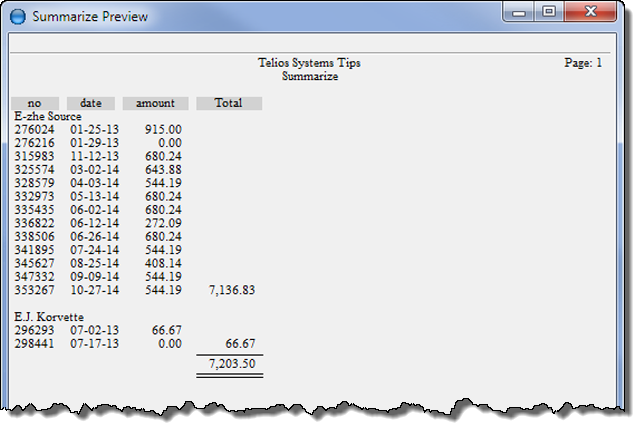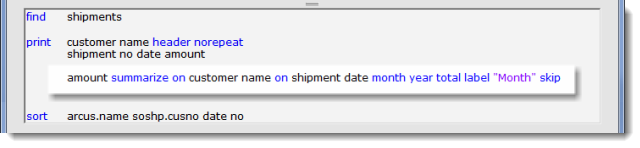# Print — Summarize

### Numeric summarize

Enough talking. Here is the first example:• amount is the target field to summarize
• summarize on customer name — customer name is the filter. When the customer name changes the amount will print.
• total — prints a grand total at the end of the report.
• skip — blank line after amount prints.
• label “Total” — column label.

and the result:next we will add a date filter:• on shipment date month year
• this will add a subtotal for each month of each year for each customer.
• total — prints a grand total at the end of the report.
• skip — prints a blank line after the summarize line prints.Page 1 — summarizePage 2 — summarize syntaxPage 3 — numeric summarizePage 4 — date summarizePage 5 — expression summarizePage 6 — text summarize

Next – date summarize >